UPSC  >  Types of Questions in Letter Series

# Types of Questions in Letter Series - CSAT Preparation - UPSC

 Table of contentsType - 1Type - 2Type - 3Type - 4## Type - 1

### To find out the position of the letter according to the given condition.

Note: If we are moving to the left hand side of any letter that means positions are decreasing and if we are moving towards right hand side of the series that means Positions of the letters are increasing.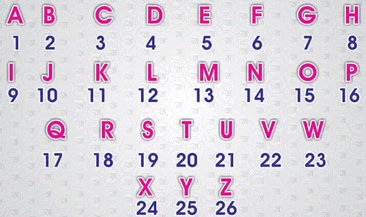If the first half and second half of the alphabet series are Arranged in reverse order, then find out the answers to the given questions:

Q.1. Which letter is 5th to the right of the 12th letter from the left-hand side of the series?
Ans. 5th to the right of 12th letter from left means 12+5 = 17th letter of the series.
Because series is in reverse order for that the trick is to:

• Add the first and last position of the group in which this position falls.
• Deduct the given position from the sum we will get the position of the same letter in the real series.

Hence, the letter 17th falls in the 2nd half which starts from position 14th and ends on 26th.
∴ 14 + 26 = 40, 40 - 17 = 23
which means the letter which is in 17th position in the given series is 23rd in the real series. Hence, the letter is “W”.

Q.2. Which letter is 5th to the left of the 12th letter from the left-hand side on the series?
Ans. 5th to the left of 12th letter from left means, 12 – 5 = 7th letter of the serries
Which falls in the first half of the series which starts from position 1st and ends on 13th.
∴ 1 + 13 = 14, 14 – 7 = 7th letter i.e.“g” in the real series.
(Position is same even in reversed series, because letter 7 is in the middle of the series, and its position will not be Changed even after letter get reversed)

Q.3. Which letter is the 5th to the left of the 12th letter from the right-hand side of the series?
Ans. 5th to the left of the 12th letter from the right.
In this case first of all we will convert the 12th letter from right to its position from left. (27 – 12 = 15)
⇒ We have to find the 5th letter to the left of the 15th letter from the left.
Hence,  15 – 5 = 10th letter, that falls in first half of the series.
∴ 1 + 13 = 14,   14 – 10 = 4th letter i.e. “d”.

Q.4. Which letter is 5th to the right of the 12th letter from the right-hand side of the series?
Ans. 5th to the right of the 12th letter from the right end.
► 12th from the right end means 27-12 = 15th from left.
⇒ 15 + 5 = 20th letter, which falls in the second half of the series.
∴ 14 + 26 = 40,  40 – 20 = 20th letter i.e. “t”.
(because position 20 is in the middle of the second half of the series).

## Type - 2

### To find out the next letter of the series.

Find The Letter In Place Of Question Mark (?) In The Series Given Below:
Q.1. B, F, ?, N, R
(a) G
(b) K
(c) J
(d) L
(e) None of The Above
Ans. (c)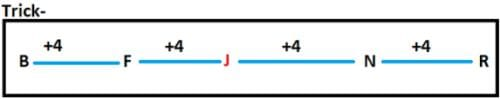Q.2. P, ?, J, G, D, A
(a) Q
(b) N
(c) K
(d) M
(e) None of The Above
Ans. (d)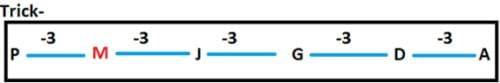Q.3. ?, E, G, J, N
(a) A
(b) B
(c) D
(d) Z
(e) None of The Above
Ans. (c)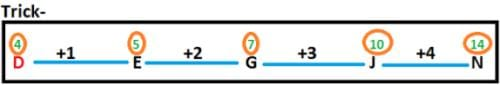Q.4. X, F, Y, G, ?, H
(a) Z
(b) A
(c) B
(d) Y
(e) None of The Above
Ans. (a)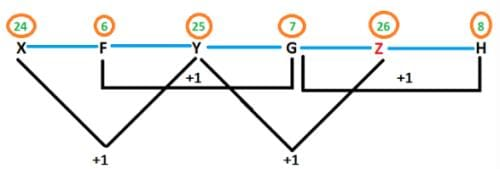Q.5. B, B, A, D, ?, F
(a) B
(b) A
(c) Z
(d) C
(e) None of The Above
Ans. (c)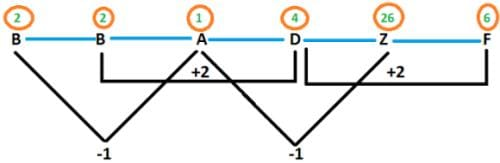## Type - 3

### Fill in the blank series

These types of series consist of small letters which follow a specific pattern or series. Some spaces are left blank in between the series given. We have to fill in the blanks from the given options to make a pattern.
This can be clearly explained by the following examples:

Example 1.   a _ c a a _ b c c _ a a b bb _ c c
(a) bbca
(b) abac
(c) bbac
(d) bacb

Ans. (b)
Explanation. We can solve questions of these types by following steps:

• Check out the total number of positions in the statement which is 18 here.
• Now,18 can be divided by 2, 3, 6, 9 if the pattern here is of groups of equal size.
• We can’t divide them into the group of 2 or 3 letters here because of repeated letters in the last of the series.
• If we divide them into a group of 6 or 9 letters each then also we are not getting a particular pattern here.
• Hence, groups here are of different sizes.
• Considering this part _ a a b bb _ c c, we can see that letter “b” comes thrice here, which means the letter “a” and “c” also could be thrice and if we fill this with a and c respectively, we will get that – a aa b bb c cc .
• That means, in the previous group letters are repeated twice- a a _ b c c. if we fill it with “b”.
• So, groups here are formed by all the three letters first one time then twice, and at last thrice.
• By looking at the options we will get the option (c) – bbac is our answer.

Example 2.  a _ b b a a _ b a a _ b a a b _ a a b
(a) abab
(b) baba
(c) abbb
(d) babb

Ans. (c)
Explanation.
We can solve this by following steps:

• If we look at the total no. of positions that are 19.
• Because, 19 is a prime number that means we can’t divide this, so we can use either 18 or 16 letters to divide into groups.
• If we are taking 18 letters, we can divide them into groups of 3, 6, and 9 letters from which we will get optimum numbers and size of the group if we divide by 6. But, it will also not lead to a particular pattern.
• If we are taking 16, we can divide it into groups of 2, 4, and 8 letters of which we will get the optimum number and size of the group if we divide it by 4. therefore,-a _ b b a a _ b a a _ b a a b _ a a b,
• Now, we will get same group of same size, therefore pattern is - a a b b a a b b a a b b a a b b a a b.
• Thus, we will get with the help of option (c) – abbb.

Example 3. a _ b c a a _ _ aa b _ a a _ c a a _ c
(a) accbbb
(b) acbcbb
(c) cbcbba
(d) abccbb

Ans. (d)
Explanation.
We can solve this by following steps:

• Total no. of positions here are 20 which can be divided by 2, 4, 5 and 10.
• To make an optimum group of optimum size either we can divide it by 4 or by 5.
• So, if we make groups of 4 letters each: a _ b c a a _ _ aa b _ a a _ c a a _ c,
We will get identical groups.
• Therefore, the pattern is: a a b c a a b c a a b c a a b c a a b c
• Thus, we will get with the help of option(d) – abccbb.

## Type - 4

### Miscellaneous Questions

In the exam one can also ask questions to make a meaningful word, or by arranging letters in ascending or descending order, or by getting the same gap in between two letters as in the alphabet series.
This can be clearly explained by the following examples:

Example 1. If the letters of the word “ BEAUTIFUL” are arranged in Ascending/increasing order. How many letters are there whose position will not change?
(a) 4
(b) 3
(c) 1
(d) 2

Ans. (c)
Explanation.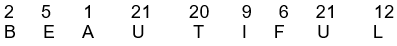In ascending order: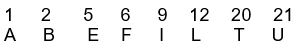As we can see only letter “U” is on the same position after rearrangement. Therefore, answer is option – (c).

Example 2. How many pairs are there in the word “BEAUTIFUL”, which have the same number of letters between them as in the alphabet series?
(a) 1
(b) 4
(c) 3
(d) none

Ans. (b)
Explanation.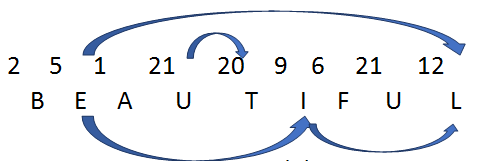Therefore, ans is option - (b)

Example 3. With the help of letters “ E , S, R , O” each letter used
Ones, how many meaningful words can be formed?
(a) 1
(b) 2
(c) 3
(d) none

Ans. (a)
Explanation. With the help of these letters only one word is formed: ROSE.
Hence, the answer is option – (a)

Note:

These types of questions are just to check your General intelligence.

Example 4. With the help of the letters “A, D, E, R” each letter used
Ones, how many meaningful words can be formed?
(a) 2
(b) 3
(c) more than 3
(d) none

Ans. (c)
Explanation. With the help of these letters words formed are: READ, DEAR, DARE, RADE
Hence, the answer is option- (c).

The document Types of Questions in Letter Series | CSAT Preparation - UPSC is a part of the UPSC Course CSAT Preparation.
All you need of UPSC at this link: UPSC

## CSAT Preparation

197 videos|151 docs|200 tests

## FAQs on Types of Questions in Letter Series - CSAT Preparation - UPSC

 1. What are the different types of questions in a letter series?Ans. The different types of questions in a letter series include Type 1, Type 2, Type 3, and Type 4. These types are based on the pattern or rule followed in the series of letters.
 2. What is Type 1 question in a letter series?Ans. Type 1 question in a letter series usually involves finding the missing letter(s) in a given series. The series follows a specific pattern, and the task is to identify the pattern and determine the missing letter(s) accordingly.
 3. Explain Type 2 question in a letter series.Ans. Type 2 question in a letter series requires identifying the letter(s) that comes in a specific position in the given series. The series follows a certain pattern or rule, and the task is to find the letter(s) at a particular position.
 4. How can Type 3 question in a letter series be solved?Ans. Type 3 question in a letter series involves finding the wrong or incorrect letter(s) in a given series. The series follows a pattern, but one or more letters deviate from the pattern. The task is to identify the incorrect letter(s) and determine the correct series.
 5. What is the concept behind Type 4 question in a letter series?Ans. Type 4 question in a letter series requires finding the next letter(s) in the given series based on a specific pattern or rule. The task is to recognize the pattern and determine the letter(s) that should come next in the series.

## CSAT Preparation

197 videos|151 docs|200 testsExplore Courses for UPSC exam### How to Prepare for UPSC

Read our guide to prepare for UPSC which is created by Toppers & the best Teachers
Signup to see your scores go up within 7 days! Learn & Practice with 1000+ FREE Notes, Videos & Tests.
10M+ students study on EduRev
Track your progress, build streaks, highlight & save important lessons and more!
Related Searches

,

,

,

,

,

,

,

,

,

,

,

,

,

,

,

,

,

,

,

,

,

;